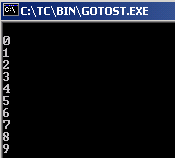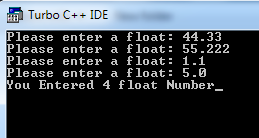### Factorial in C

Write a program in c to find the factorial of a number

#include<stdio.h>
int main(){
int i=1,f=1,num;
printf("Enter a number: ");
scanf("%d",&num);
while(i<=num){
f=f*i;
i++;
}
printf("Factorial of %d is: %d",num,f);
return 0;
}
Sample output:
Enter a number: 5
Factorial of 5 is: 120

### Calculate the Series

visit the problem source

#include <stdio.h>
#include<conio.h>
int main ()
{
int n,i,s,p,j;
clrscr();
scanf("%d",&n);
p=1;
for (i=1;i<=n;i++)
{
s=0;
for (j=1;j<=i;j++)
{
s=s+i;
}
p*=s;
}
printf("\n The value of p is =%d",p);
getch();
return 0;
}

sample input/output:

input: 4
output: The value of p is =576

### Find Ascii Values

This prints out all acsii values

``````void main()
{
int i;
i=0;
do
{
printf("%d %c \n",i,i);
i++;
}
while(i<=255);
}``````

This prints out the acsii value for a given character:
`` ``
``````void main()
{
int e;
char ch;
clrscr();
printf("\n Enter a character : ");
scanf("%c",ch);
e=ch;
printf("\n The ASCII value of the character is : %d",e);
getch();
}``````

### Goto in C

``` #include <stdio.h> #include <conio.h> int main() {   int n = 0;   loop: ;     printf("\n%d", n);   n++;   if (n<10) {   goto loop;   }   getch();   return 0; }```

``

### Float Array

#include <stdio.h>
#include<conio.h>
int main()
{
int i;
float fArray;
clrscr();
for (i = 0; i < 4; i++)
{
scanf("%f", fArray[i]);
}
getch();
return 0;
}

output:### Matrix in C

Write a program for the addition of a 2*2 matrix.

Source Code:

### Function in C

Write a program to show the advantage of using function in a program

Source Code:

### Break and Continue in C

Differentiate between break and continue using C source code

Source code:

### Sum the selected digits

If a four digit integer number is input through the keyboard then write a c program to find the sum of first and last digit.

Sample Input: 3254
Output: 7
Hints: 3(1st digit)+4(last digit)=7

Source Code: